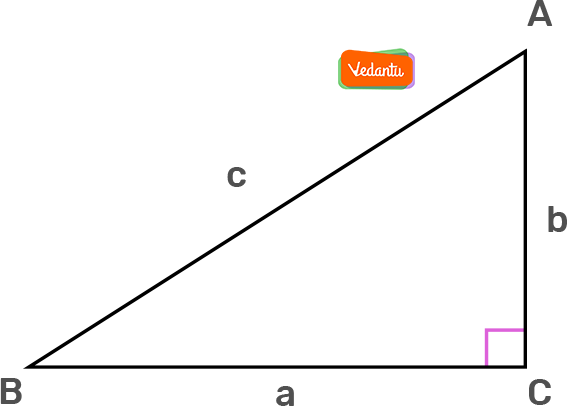# Sec 60Bookmark added to your notes.
View Notes

## Sec 60 Value

The word Trigonometry is derived from two roots i.e. trignon means "triangle", and metric means "measure". The study of trigonometry is based on the study of measurement of the triangle. What can we measure in a triangle? We can simply measure the length of the sides, angles of a triangle, and the area included in a given triangle.

We can measure the angles and length of the sides of a right-angled triangle with the help of the trigonometric ratios. Sine, cosine, tangent, secant, cosecant, and cotangent are six trigonometric ratios that represent the relation between the angles, and the length of the sides of a right-angled triangle. The ratio of the three sides of a right-angled triangle in terms of any of its acute angles is considered as the trigonometry ratio of that specific angle.

In this article, we will briefly discuss sec 60, and how to derive the secant 60 value.

 What is the Value of Sec 60?The Value of Sec 60 is 2

### Sec 60 Degree Value

As we know sec 60 degree or sec 60 value is 2. Let us first know the importance of the secant function in trigonometry, before discussing how the sec 60 value is derived geometrically.

The secant function is the opposite of the cosine function in trigonometry. In a right-angled triangle, the secant of an angle is defined as the ratio of the length of the hypotenuse side to the length of the adjacent side. In the formula, the word secant is abbreviated as sec.

In the above right-angled triangle, the formula for secant θ or sec θ is derived as

Sec θ = Hypotenuse/ Adjacent

Or

Sec θ = C / B

### Sec 60 Degree Value Derivation

To derive the value of sec 60 degree, let us consider an equilateral triangle ABC. As we know each angle of an equilateral triangle is 60°, accordingly, ∠A = ∠B = ∠C.

Construction: Drawn the perpendicular line AD from A to the side BC.

In ΔABD and ΔACD

∠B = ∠C.

AB = AC ( As we know all the sides of an equilateral triangle are equal)

AD = AC( Common side)

Hence, by the Angle-Side Angle theorem, we can say that

ΔABD ≅ ΔACD

So, BD = DC, and ∠BAD = ∠ CAD ( By CPCT)

It is seen that ABD is a right triangle, right angled at D with

∠BAD = 30°, and ∠ ABD = 60°

To determine the trigonometric ratios, we need to determine the length of the sides of the triangle. So, let us assume the side AB = 2a, and BD = BC/2 = a.

Let us consider ΔABD to determine the value of cos 60 in which AB = 2a, and BD = a. Accordingly,

Cos θ = Adjacent Side/ Hypotenuse Side

Cos 60° = BD/AB

Cos 60° = a/2a = ½

As we know, the secant function is the inverse function of the cosine function, it becomes:

Sec 60° = 1/ cos 60°

Sec 60° = 1/ (½) = 2

Hence, the value of Sec 60° = 2

Similarly, we can derive the other degrees of sec value like 0°, 30,° 45°, 90°, 180°, 270°, and 360°.

### Solved Example

1. Calculate the value of sec 300°

Solution:

Sec 300° = Sec (360 - 60)°

= Sec 60°, As we know, sec (360° - θ ) = sec θ.

2. Find the value of tan 45 + sec 60.

Solution:

As we know, tan 45 = 1 , and sec 60 = 2

Hence, substituting the values we get:

1 + 2 = 3

Therefore, the value of  tan 45 + sec 60 = 3.

FAQ (Frequently Asked Questions)

1. What are the Trigonometric Functions?

Ans: Trigonometric functions relate the angles in a right triangle to the ratio of its sides. It implies that the relation between the angles and sides of a right triangle is derived by trigonometric functions. Trigonometric functions are also known as a circular function. Sine, cosine, tangent, secant, cosecant, and cotangent are six trigonometric functions.

2. What is the Secant Function Reciprocal of?

Ans: The secant function is the reciprocal or inverse of the cosine function. The cosine function is defined as the relation between the adjacent side, and the hypotenuse side of a right triangle in terms of the angle formed between the adjacent and hypotenuse side. Hence, we can say the secant function is defined as the relation between the hypotenuse side, and the adjacent side of a right triangle in terms of the angle formed between the hypotenuse and adjacent side.In the above right triangle,

Cos θ = Adjacent / Hypotenuse

Whereas,

Sin θ = Hypotenuse / Adjacent

3. What is the Value of Sec 60 Degree and Cos 60 Degree?

Ans: As we know, the secant is the reciprocal of cosine. Accordingly, the value of sec 60 degrees is 2 whereas the value of cos 60 degrees is ½.

4. What are the Basic  Trigonometric Functions?

Ans: Sine, cosine, and tangent are the three prime or basic trigonometric functions that are widely used. Secant, Cosecant, and Cotangent are three additional trigonometric functions that are derived from primary function, and are not widely used.In a right triangle, if we consider the angle, and represent the side concerning the angle, then ‘a’ is the length of the adjacent side, ‘b’ is the length of the opposite side,  and  ‘c’ is the length of the hypotenuse side. Accordingly, the basic trigonometric functions are expressed as:

Sin = Adjacent/ Hypotenuse = BC/AC = a/c

Cos = Opposite /Hypotenuse = AC/AB = b/c

Tan =  Opposite / Adjacent =  AC/BC = b/a

Value of Cos 60Sec 90Sec 0Sec 30Table of 60 - Multiplication Table of 60Cos 60 degreesTan 60 DegreesSin 60 DegreesFactors of 60Sec 30 Degrees